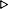ToC DocOverview CGDoc RelNotes Index PermutedIndex
Allegro CLANSI Common Lisp22 Printer22.4 Dictionary of Printer

Value Type:
a generalized boolean.

Initial Value:
false.

Description:
If *print-readably* is true, some special rules for printing objects go into effect. Specifically, printing any object O1 produces a printed representation that, when seen by the Lisp reader while the standard readtable is in effect, will produce an object O2 that is similar to O1. The printed representation produced might or might not be the same as the printed representation produced when *print-readably* is false. If printing an object readably is not possible, an error of type print-not-readable is signaled rather than using a syntax (e.g., the "#<" syntax) that would not be readable by the same implementation. If the value of some other printer control variable is such that these requirements would be violated, the value of that other variable is ignored.

Specifically, if *print-readably* is true, printing proceeds as if *print-escape*, *print-array*, and *print-gensym* were also true, and as if *print-length*, *print-level*, and *print-lines* were false.

If *print-readably* is false, the normal rules for printing and the normal interpretations of other printer control variables are in effect.

Individual methods for print-object, including user-defined methods, are responsible for implementing these requirements.

If *read-eval* is false and *print-readably* is true, any such method that would output a reference to the "#." reader macro will either output something else or will signal an error (as described above).

Examples:
``` (let ((x (list "a" '\a (gensym) '((a (b (c))) d e f g)))
(*print-escape* nil)
(*print-gensym* nil)
(*print-level* 3)
(*print-length* 3))
(write x)
(terpri)
(write x)
:done))(a a G4581 ((A #) D E ...))("a" |a| #:G4581 ((A (B (C))) D E F G)):DONE

;; This is setup code is shared between the examples
;; of three hypothetical implementations which follow.
(setq table (make-hash-table))#<HASH-TABLE EQL 0/120 32005763>
(setf (gethash table 1) 'one)ONE
(setf (gethash table 2) 'two)TWO

;; Implementation A
Error: Can't print #<HASH-TABLE EQL 0/120 32005763> readably.

;; Implementation B
;; No standardized #S notation for hash tables is defined,
;; but there might be an implementation-defined notation.#S(HASH-TABLE :TEST EQL :SIZE 120 :CONTENTS (1 ONE 2 TWO))#<HASH-TABLE EQL 0/120 32005763>

;; Implementation C
;; Note that #. notation can only be used if *READ-EVAL* is true.
;; If *READ-EVAL* were false, this same implementation might have to
;; signal an error unless it had yet another printing strategy to fall
;; back on.#.(LET ((HASH-TABLE (MAKE-HASH-TABLE)))(SETF (GETHASH 1 HASH-TABLE) ONE)(SETF (GETHASH 2 HASH-TABLE) TWO)HASH-TABLE)#<HASH-TABLE EQL 0/120 32005763>
```